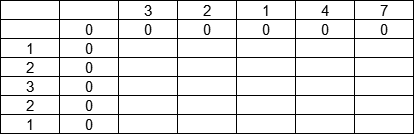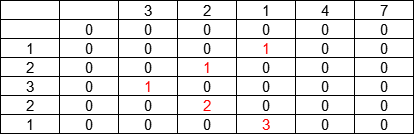# LeetCode 题解：718. 最长重复子数组，动态规划，JavaScript，详细注释Lee Chen1. 假设A的长度为mB的长度为n。我们可以创建一个(m+1)*(n+1)矩阵dp，为避免递推第一个值时索引出现负数，dp矩阵要创建第一行和第一列的初始值0，避免边界判断。

AB排列如下图：1. 对于A的任意索引i - 1B的任意索引j - 1，如果A[i - 1] === B[j - 1]，当前子数组长度只需要在之前已知的公共子数组长度上加 1。如果不相等，当前统计的长度就为 0.

2. 状态转移方程如下：

if (A[i - 1] === B[j - 1]) {  dp[i][j] = dp[i - 1][j - 1] + 1;} else {  dp[i][j] = 0}

/** * @param {number[]} A * @param {number[]} B * @return {number} */var findLength = function (A, B) {  const m = A.length; // 缓存A长度  const n = B.length; // 缓存B长度  let dp = new Array(m + 1); // 创建(m+1)*(n+1)矩阵用于递推  let max = 0; // 保存公共子数组最大长度  // 为矩阵填入初始值，全部都为0  for (let i = 0; i <= m; i++) {    dp[i] = new Array(n + 1).fill(0);  }  // 遍历A和B的每一个元素，统计公共子数组的长度  // 为避免i为0时，i-1为负数，因此从1开始递推  for (let i = 1; i <= m; i++) {    for (let j = 1; j <= n; j++) {      // 只有两个元素相等时，才需要计算，否则默认为0      if (A[i - 1] === B[j - 1]) {        // 当前公共子数组长度，在已有的长度上加1        dp[i][j] = dp[i - 1][j - 1] + 1;        // 最大值不会向前递推，每次都需要更新缓存        max = Math.max(dp[i][j], max);      }    }  }  return max;};1. 由于dp[i][j]只与dp[i - 1][j - 1]，也就是左上角的状态有关，因此dp矩阵可以优化成一个数组。

/** * @param {number[]} A * @param {number[]} B * @return {number} */var findLength = function (A, B) {  const m = A.length; // 缓存A长度  const n = B.length; // 缓存B长度  let dp = new Array(n + 1).fill(0); // 创建(m+1)*(n+1)矩阵用于递推  let max = 0; // 保存公共子数组最大长度  // 遍历A和B的每一个元素，统计公共子数组的长度  // 为避免i为0时，i-1为负数，因此从1开始递推  for (let i = 1; i <= m; i++) {    // 由于j的状态只与j-1的状态有关，因此必须从后往前推导，否则j-1的值在计算前会被清空    for (let j = m; j >= 1; j--) {      // 只有两个元素相等时，才需要计算，否则默认为0      if (A[i - 1] === B[j - 1]) {        // 当前公共子数组长度，在已有的长度上加1        dp[j] = dp[j - 1] + 1;        // 最大值不会向前递推，每次都需要更新缓存        max = Math.max(dp[j], max);      } else {        // 元素不相等时，公共子数组长度为0        dp[j] = 0;      }    }  }  return max;};## 评论